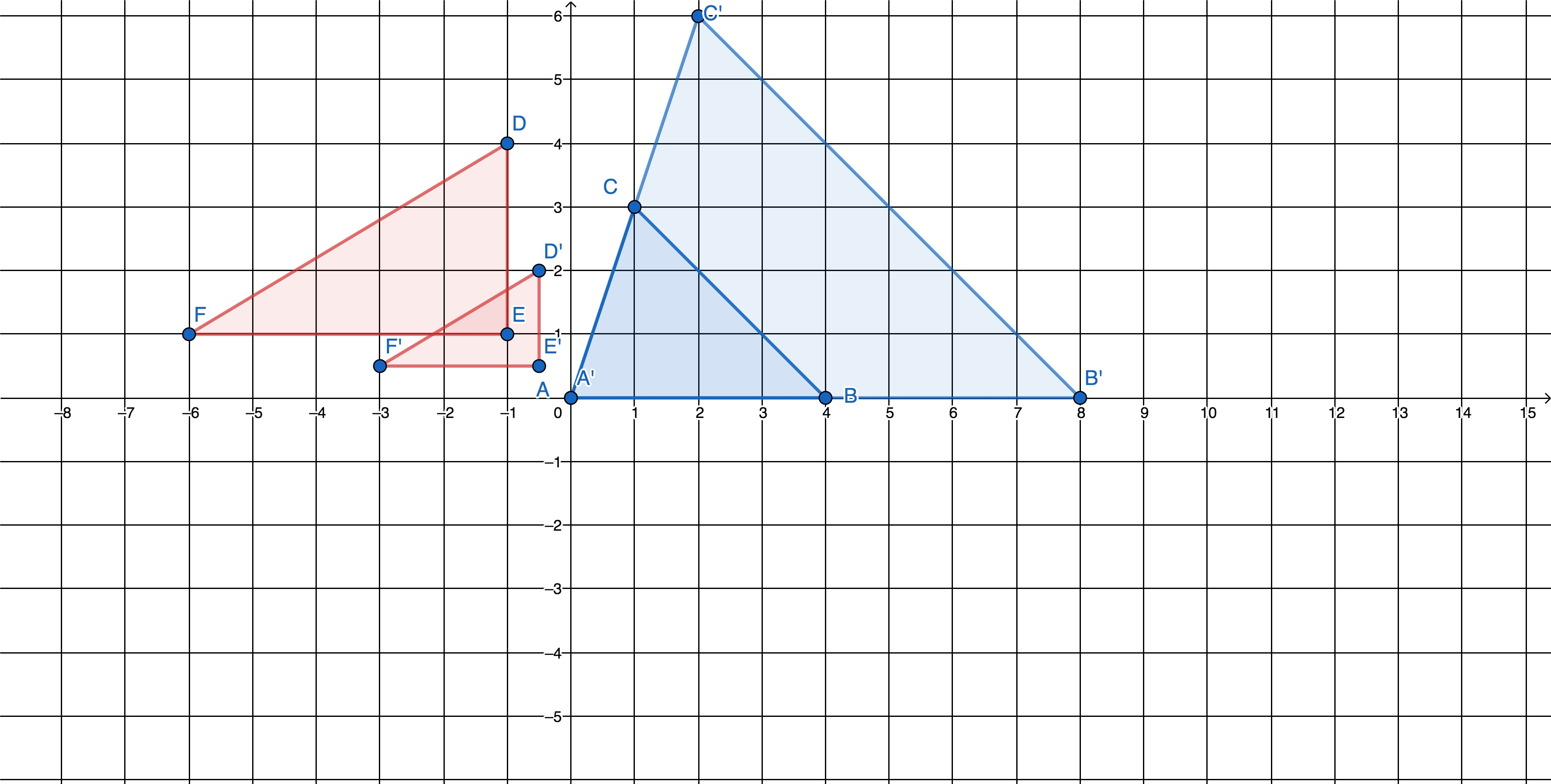# GEOM 3 | Lesson 3 | Watch (Similarity)# Similarity

What must be true if the figures are similar?

Below are similar triangles.  Notice the coordinates of the red similar triangles, $$DEF$$ and $$D’E’F’$$.  How do they compare?  Now look at the blue similar triangles, $$ABC$$ and $$A’B’C’$$.  How did the coordinates change from the original (preimage) to the image?For more on similar figures watch the folllowing on Learn Zillion.  Use the code  6MX8PG8 to sign into the video.  When you finish, come back to this page.

https://learnzillion.com/assignments/6MX8PG8

We know that the corresponding angles are congruent and the corresponding sides are in proportion in similar triangles.    If we have two triangles, how can we determine whether the are similar, without first proving all the corresponding angles are congruent and the sides are in proportion?

Theorems of Similar Triangles – AA, SAS & SSS – MrEMathVideos

Describe the following, using a figure to demonstrate.

1) Similarity

2) AA Similarity.

3) SSS Similarity.

4) SAS Similarity.